Updating search results...

# 766 Results

View
Selected filters:
• Numbers and Operations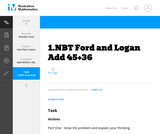Unrestricted Use
CC BY
Rating
0.0 stars

This is a task from the Illustrative Mathematics website that is one part of a complete illustration of the standard to which it is aligned. Each task has at least one solution and some commentary that addresses important aspects of the task and its potential use.

Subject:
Numbers and Operations
Material Type:
Activity/Lab
Provider:
Illustrative Mathematics
Provider Set:
Illustrative Mathematics
Author:
Illustrative Mathematics
08/06/2015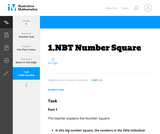Unrestricted Use
CC BY
Rating
0.0 stars

This is a task from the Illustrative Mathematics website that is one part of a complete illustration of the standard to which it is aligned. Each task has at least one solution and some commentary that addresses important aspects of the task and its potential use.

Subject:
Numbers and Operations
Material Type:
Activity/Lab
Provider:
Illustrative Mathematics
Provider Set:
Illustrative Mathematics
Author:
Illustrative Mathematics
08/06/2015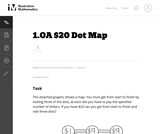Unrestricted Use
CC BY
Rating
4.0 stars

This problem helps students practice adding three numbers whose sum are 20 or less.

Subject:
Mathematics
Numbers and Operations
Material Type:
Activity/Lab
Provider:
Illustrative Mathematics
Provider Set:
Illustrative Mathematics
Author:
Illustrative Mathematics
09/10/2012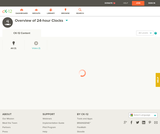Conditional Remix & Share Permitted
CC BY-NC-SA
Rating
0.0 stars

This short video and interactive assessment activity is designed to give fourth graders an overview of 24-hour clocks.

Subject:
Mathematics
Numbers and Operations
Material Type:
Assessment
Interactive
Lecture
Provider:
CK-12 Foundation
Provider Set:
CK-12 Elementary Math
03/05/2015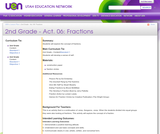Educational Use
Rating
4.0 stars

In this lesson students will explore the concept of fractions.

Subject:
Arts and Humanities
Numbers and Operations
Material Type:
Lesson Plan
Provider:
Utah Education Network
10/22/2013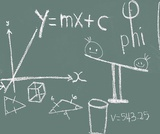Unrestricted Use
CC BY
Rating
0.0 stars

This is a short lesson plan for discussing/reviewing order of operations with technology integration.

Subject:
Educational Technology
Elementary Education
Mathematics
Numbers and Operations
Material Type:
Activity/Lab
Author:
Julia Roberts
03/06/2020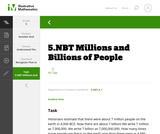Unrestricted Use
CC BY
Rating
0.0 stars

This is a task from the Illustrative Mathematics website that is one part of a complete illustration of the standard to which it is aligned. Each task has at least one solution and some commentary that addresses important aspects of the task and its potential use.

Subject:
Numbers and Operations
Material Type:
Activity/Lab
Provider:
Illustrative Mathematics
Provider Set:
Illustrative Mathematics
Author:
Illustrative Mathematics
08/06/2015Unrestricted Use
CC BY
Rating
0.0 stars

This is a task from the Illustrative Mathematics website that is one part of a complete illustration of the standard to which it is aligned. Each task has at least one solution and some commentary that addresses important aspects of the task and its potential use.

Subject:
Numbers and Operations
Material Type:
Activity/Lab
Provider:
Illustrative Mathematics
Provider Set:
Illustrative Mathematics
Author:
Illustrative Mathematics
08/06/2015
Remix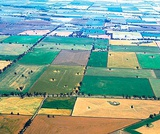Conditional Remix & Share Permitted
CC BY-NC
Rating
0.0 stars

Students use a geometric model to investigate common factors and the greatest common factor of two numbers.Key ConceptsA geometric model can be used to investigate common factors. When congruent squares fit exactly along the edge of a rectangular grid, the side length of the square is a factor of the side length of the rectangular grid. The greatest common factor (GCF) is the largest square that fits exactly along both the length and the width of the rectangular grid. For example, given a 6-centimeter × 8-centimeter rectangular grid, four 2-centimeter squares will fit exactly along the length without any gaps or overlaps. So, 2 is a factor of 8. Three 2-centimeter squares will fit exactly along the width, so 2 is a factor of 6. Since the 2-centimeter square is the largest square that will fit along both the length and the width exactly, 2 is the greatest common factor of 6 and 8. Common factors are all of the factors that are shared by two or more numbers.The greatest common factor is the greatest number that is a factor shared by two or more numbers.Goals and Learning ObjectivesUse a geometric model to understand greatest common factor.Find the greatest common factor of two whole numbers equal to or less than 100.

Subject:
Numbers and Operations
Material Type:
Lesson Plan
Author:
Angela Vanderbloom
07/05/2018
RemixConditional Remix & Share Permitted
CC BY-NC
Rating
0.0 stars

Lesson OverviewStudents use a geometric model to investigate common multiples and the least common multiple of two numbers.Key ConceptsA geometric model can be used to investigate common multiples. When congruent rectangular cards with whole-number lengths are arranged to form a square, the length of the square is a common multiple of the side lengths of the cards. The least common multiple is the smallest square that can be formed with those cards.For example, using six 4 × 6 rectangles, a 12 × 12 square can be formed. So, 12 is a common multiple of both 4 and 6. Since the 12 × 12 square is the smallest square that can be formed, 12 is the least common multiple of 4 and 6.Common multiples are multiples that are shared by two or more numbers. The least common multiple (LCM) is the smallest multiple shared by two or more numbers.Goals and Learning ObjectivesUse a geometric model to understand least common multiples.Find the least common multiple of two whole numbers equal to or less than 12.

Subject:
Numbers and Operations
Material Type:
Lesson Plan
Author:
Angela Vanderbloom
07/07/2018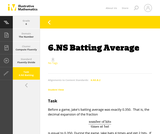Unrestricted Use
CC BY
Rating
0.0 stars

This is a task from the Illustrative Mathematics website that is one part of a complete illustration of the standard to which it is aligned. Each task has at least one solution and some commentary that addresses important aspects of the task and its potential use.

Subject:
Numbers and Operations
Material Type:
Activity/Lab
Provider:
Illustrative Mathematics
Provider Set:
Illustrative Mathematics
Author:
Illustrative Mathematics
08/06/2015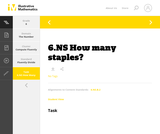Unrestricted Use
CC BY
Rating
0.0 stars

This is a task from the Illustrative Mathematics website that is one part of a complete illustration of the standard to which it is aligned. Each task has at least one solution and some commentary that addresses important aspects of the task and its potential use.

Subject:
Numbers and Operations
Material Type:
Activity/Lab
Provider:
Illustrative Mathematics
Provider Set:
Illustrative Mathematics
Author:
Illustrative Mathematics